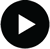## Significant Figures### Measurements, units and significant figuresVideo tutorial
In your experimental work, you will be recording a variety of experimental measurement, as well as performing some calculations using them. Measurement is at the heart of science - whether a scientific theory survives or fails depends on how well it matches up with experimental measurement. Being able to accurately measure and record experimental data are key attributes of professional scientist and ones which you will develop during the laboratory course this year.

# Reporting Measurements

 In almost all cases, reporting numerical results requires that the units are specified. There is always a space between a number and its unit. There is never a space between a unit and its prefix. There is always a space between each part of a compound unit.

These rules are commonly disregarded in the media and this leads to ambiguity. We do not ignore these rules and neither should you! They are there for very good reasons.

Examples
• One TV cook specifies that 25 g of butter is needed for a recipe and another states that 25 of butter is required". Which recipe is easier to follow?
• A measurement is reported as "10m". Since the letter "m" is used for both metres and to represent the mass, this could have two meanings. It could mean "ten metres" or it could mean "ten times the mass". You can't tell which! If it means "ten metres", it would be written as 10 m (i.e. with a space). If it means "ten times the mass", it would be written as 10m (i.e. without a space). Now you can tell which it means! Note that there is no "." after the unit. Units are not abbreviations.
• A measurement is reported as "10 ms-1" - does this mean "ten per millisecond" or does it mean "ten metres per second"? Rules (3) and (4) make it clear that it means "ten per millisecond".
• A measurement is reported as "10 m s-1" - does this mean "ten per millisecond" or does it mean "ten metres per second"? Rules (3) and (4) make it clear that it means "ten metres per second".

# Significant figures

We have imperfect senses and we use imperfect measuring devices. All measurements we make include some uncertainty. When we use these data, it is important that we recognize what uncertainty is and do not exaggerate its precision.

The number of digits that are reported or recorded are called significant figures ("sf"): an uncertainty of one units in the right most digit is assumed.

The number of significant figures that data is known to is not given explicitly in scientific reports (and not in our exams!).
 All digits are significant, except for zeros that are not measured but are used only to position the decimal point. Make sure that the measurement has a decimal point. (Add one if necessary.) Start at the left of the number and move right until you reach the first non-zero digit. Count that digit and every one to its right as significant. Zeros that end a number and lie after or before the decimal point are significant.

Examples:
• 0.0054 L is known to 2 sf
• 103.2 s is known to 4 sf
You should take care with zeros that end a number:
• 0.020 L is known to 2 sf
• 100.20 s is known to 5 sf
• 100 mol is known to only 1 sf (rule 4)
• 100. g is known to 3 sf (rule 4)
Look at the last two examples again - they may surprise you. A terminal decimal point as in the last example means all the zeros are significant.

# Calculations and significant figures

 The least certain measurement sets the limit on the certainty for the calculation and the number of significant figures in the answer. When multiplying or dividing, the answer should contain the same number of significant figures as the measurement with the fewest significant figures. When adding or subtracting, the answer should contain the same number of decimal places as the measurement with the fewest decimal places.

Examples:
• The concentration (c) of salt in seawater is measured to be 0.564 mol L-1. The number of moles (n) of a volume (V) of 1.5 L of seawater is:
n = c × V = 0.564 × 1.5 = 0.85 mol
The calculator may show "0.846" but as the volume is only known to 2 sf, the answer is too.
• If the contents of two beakers, one containing 20.1 mL of water and one containing 30.02 mL of water, are added together the total volume of water is: V = 20.1 + 30.02 = 50.1 mL The calculator may show "50.12" mL but the volume in the first beaker is only known to 1 decimal place and so the answer is also only known to 1 decimal place.

# Scientific notstion for very large and very small numbers

Very large and very small numbers are often encountered in Chemistry. Writing them out in full can be very time consuming and can lead to text which is difficult to read. Instead, scientific notation (also called exponential notation) is used. Numbers are expressed in the form:
N x 10n
where N is a number between 1 and 10 and n is the exponent. n is positive for large and negative for small numbers.

If you are not familiar with scientific notation, quickly work through the appendix.